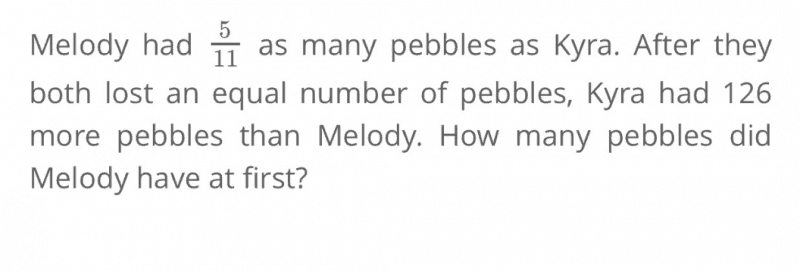# QuestionAt first, Melody had 5 units and Kyra had 11 units. Since both Melody and Kyra lost an equal number of pebbles, this means that even before they had lost their pebbles, Kyra already had 126 more pebbles than Melody.

The difference between them will always remain the same if I add or subtract an equal amount from both of them.

Therefore, the difference of 6 units between them at first is also equals to 126.

6 units = 126
1 unit = 126 ÷ 6
= 21
5 units = 21 × 5
= 105 pebbles

0 Replies 0 Likes ✔Accepted Answer